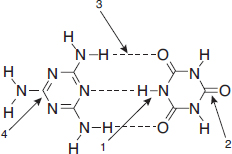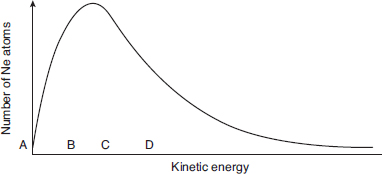# AP Chemistry Practice Test 22

### Test Information12 questions18 minutes

1. The rate of a chemical reaction is determined by

2. Using fundamental trends in electronegativity and bond strength, which of the following should be the strongest acid?

3.

 Compound Formula Normal Boiling Point, K Ethane CH3CH3 185 Ethanal CH3COH 294 Ethanol CH3CH2OH 348 Ethanoic acid CH3CO2H 391

Based on the data in the table above, which of the following substances has the lowest surface tension at -120 °C?

4.

 Ion Ionic Radius, pm O2- 66 S2- 104 Se2- 117

Based on the data in the table above, which of the following correctly predicts the strength of binary acids from weakest to strongest?

5. Zinc metal is often used to reduce substances in aqueous solutions. If zinc metal is not available, which of the following would be a reasonable and safe substitute for zinc?

6.In the diagram above, which labeled arrow is pointing toward a hydrogen bond?

7. Which would be the easiest way to burn an iron nail?

8. S(s) + O2(g) → SO2(g)

The reaction of sulfur with oxygen is written in equation form above. This equation can be interpreted in all of the following ways except

9. An experiment was performed to determine the moles of hydrogen gas formed (collected over water) when an acid reacts with magnesium metal. To do this, a piece of dry magnesium was weighed. Then 50 mL of hydrogen was collected. Next the Mg was dried to remove approximately 0.1 mL of water and weighed again to see how much Mg had reacted. The atmospheric pressure was measured as 755 torr. The volume of hydrogen was measured and converted into moles of hydrogen. Which mistake will give the smallest error in the result?

10.The graph above shows the distribution of kinetic energies of a large number of Ne atoms at 500 K. Which letter shows the average kinetic energy of this system?

11. A 25 g sample of a liquid was heated to 100 °C and then quickly transferred to an insulated container holding 100 g of water at 22 °C. The temperature of the mixture rose to reach a final temperature of 35 °C. Which of the following can be concluded?

12. Identify the Brønsted-Lowry conjugate acid–base pair in the following list.